2.2   Libraries and Clients

Each program that you have written consists of Java code that resides in a single .java file. For large programs, keeping all the code in a single file is restrictive and unnecessary. Fortunately, it is very easy in Java to refer to a method in one file that is defined in another. This ability has two important consequences on our style of programming:

• It allows us to extend the Java language by developing libraries of static methods for use by any other program, keeping each library in its own file.

• It enables modular programming, where we divide a program up into static methods, grouped together in some logical way.

Using static methods in other programs.

To refer to a static method in one class that is defined in another, you must make both classes accessible to Java (for example, by putting them both in the same directory in your computer). Then, to call a method, prepend its class name and a period separator. For example, SAT.java calls the cdf() method in Gaussian.java, which calls the pdf() method, which calls the exp() and sqrt() methods in Math.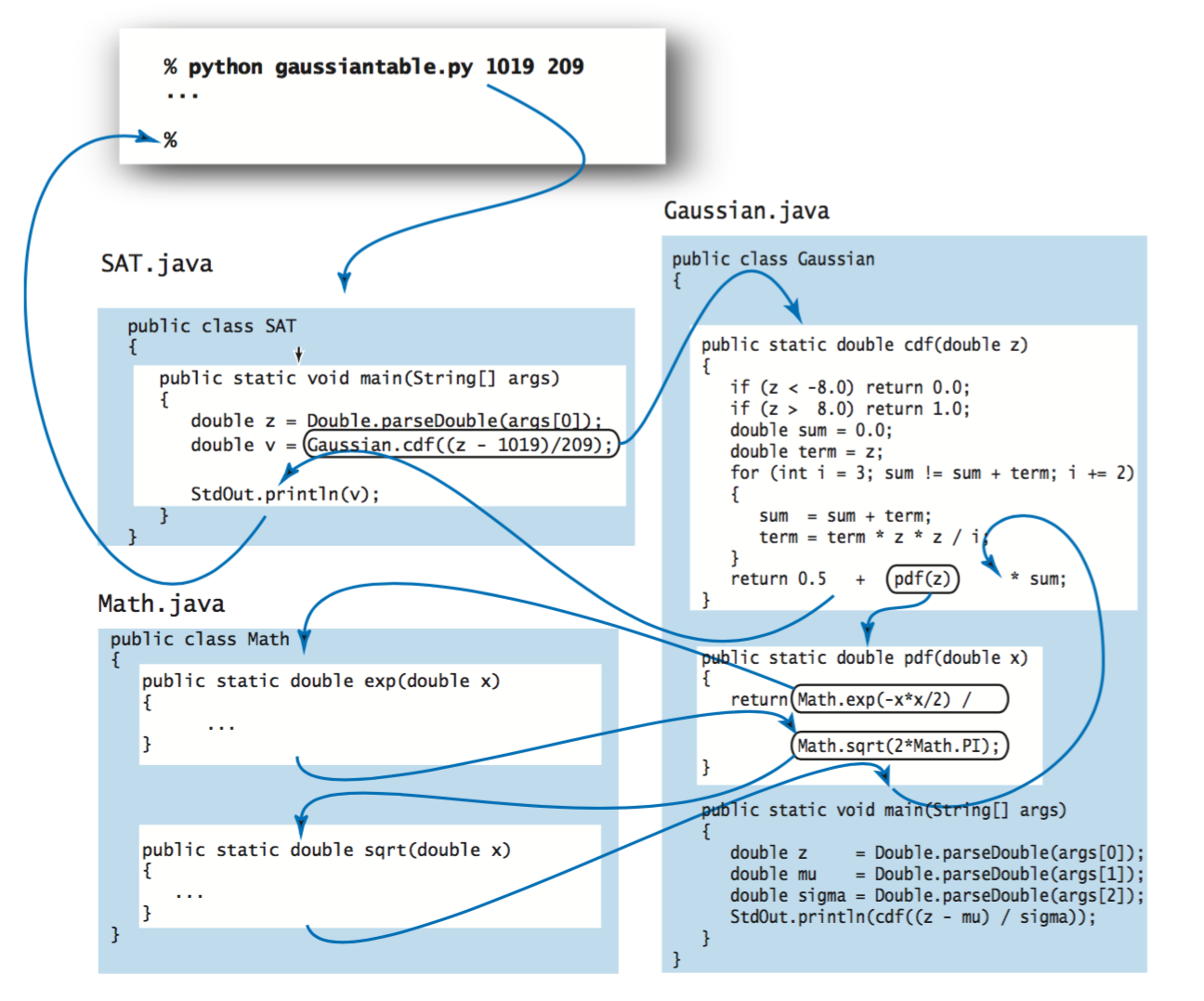We describe several details about the process.

• The public keyword. The public modifier identifies the method as available for use by any other program. You can also identify methods as private, but you have no reason to do so at this point.

• Each module is a class. We use the term module to refer to all the code that we keep in a single file. By convention, each module is a Java class that is kept in a file with the same name of the class but has a .java extension. In this chapter, each class is merely a set of static methods.

• The .class file. When you compile the program, the Java compiler makes a file with the class name followed by a .class extension that has the code of your program in a language more suited to your computer.

• Compile when necessary. When you compile a program, Java typically compiles everything that needs to be compiled in order to run the program. For example, when you type javac SAT.java, the compiler will also check whether you modified Gaussian.java since the last time it was compiled. If so, it will also compile Gaussian.

• Multiple main() methods. Both SAT.java and Gaussian.java have their own main() method. When you type java followed by a class name, Java transfers control to the machine code corresponding to the main() method defined in that class.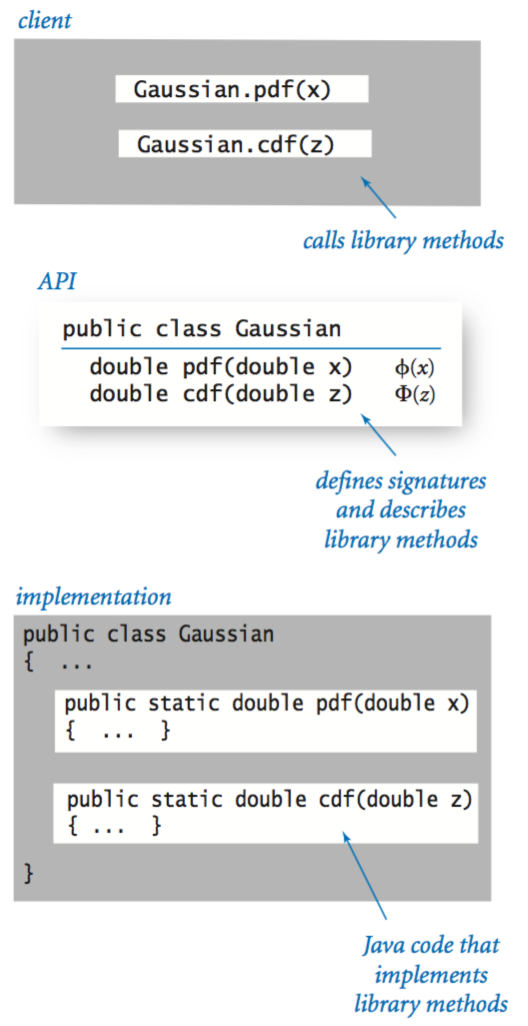Libraries.

We refer to a module whose methods are primarily intended for use by many other programs as a library.

• Clients. We use the term client to refer to a program that calls a given library method.

• APIs. Programmers normally think in terms of a contract between the client and the implementation that is a clear specification of what the method is to do.

• Implementations. We use the term implementation to describe the Java code that implements the methods in an API.

As an example, Gaussian.java is an implementation of the following API:Random numbers.

StdRandom.java is a library for generating random numbers from various distributions.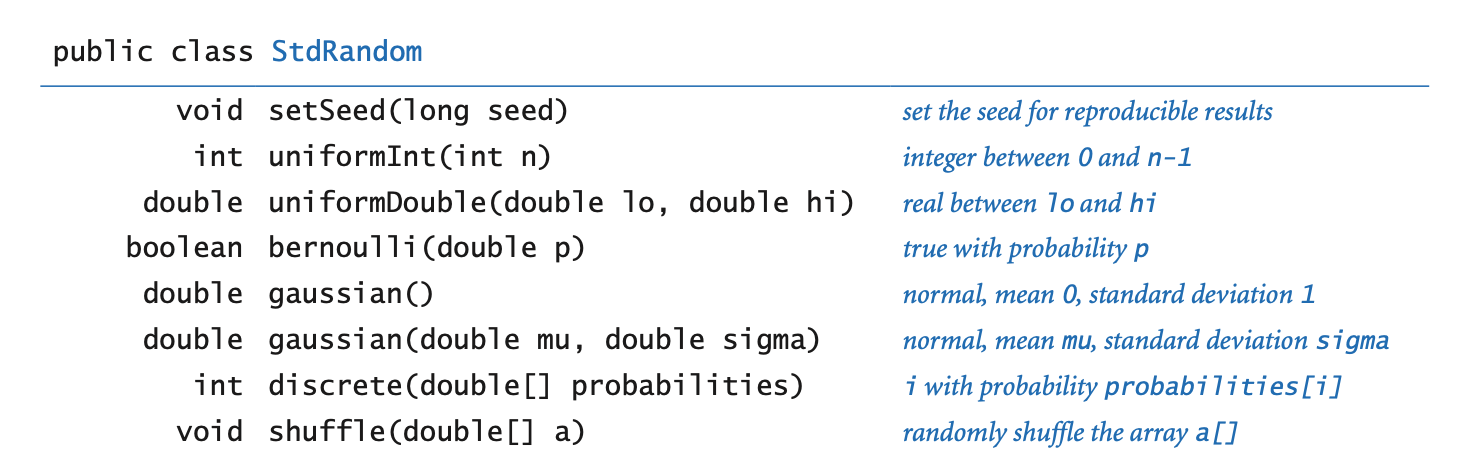Input and output for arrays.

StdArrayIO.java is a library for reading arrays of primitive types from standard input and printing them to standard output.Iterated function systems.

An Iterated function system (IFS) is a general way to produce fractals like the Sierpinski triangle or Barnsley fern. As a first example, consider the following simple process: Start by plotting a point at one of the vertices of an equilateral triangle. Then pick one of the three vertices at random and plot a new point halfway between the point just plotted and that vertex. Continue performing the same operation.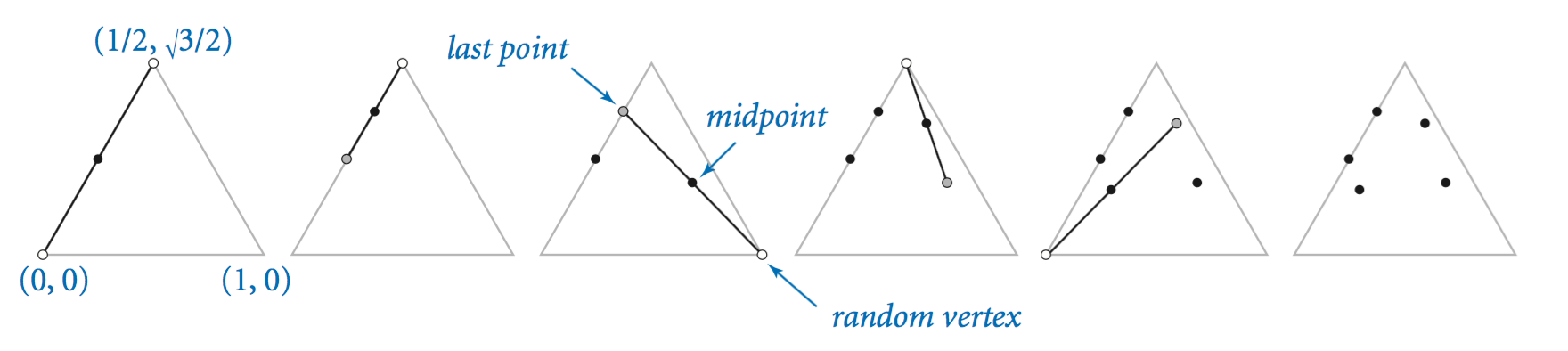Sierpinski.java simulates this process. Below are snapshots after 1,000, 10,000, and 100,000 steps.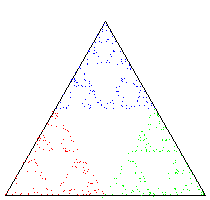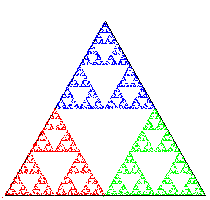IFS.java is a data-driven version program that simulates a generalization of this process. You can run it on the inputs sierpinski.txt, barnsley.txt, tree.txt, and coral.txt.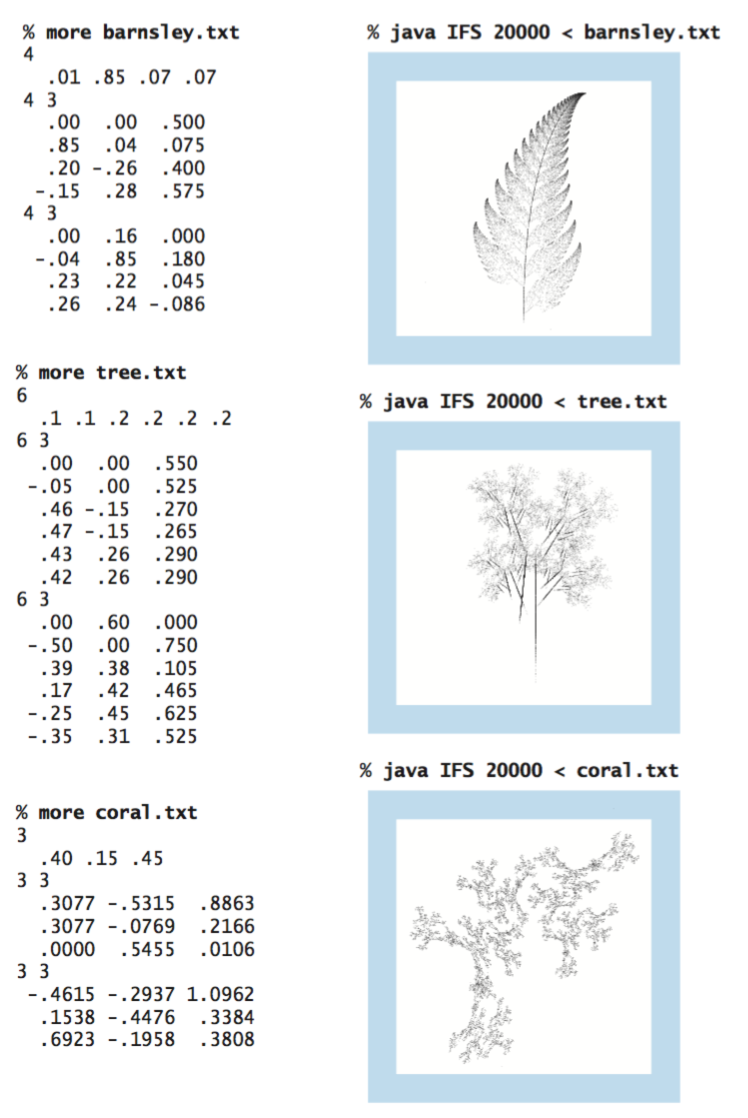Standard statistics.

StdStats.java is a library for statistical calculations and basic visualizations.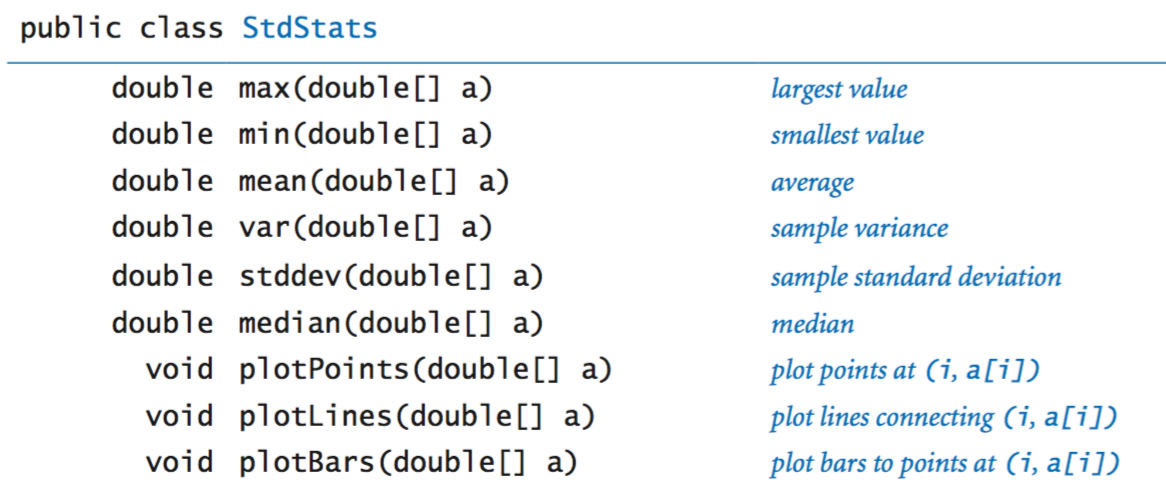Bernoulli trials.

Bernoulli.java counts the number of heads found when a fair coin is flipped n times and compares the result with the predicted Gaussian distribution function. According to the Central Limit Theorem, the resulting histogram is extremely well approximated by the Gaussian distribution with mean n/2 and variance n/4.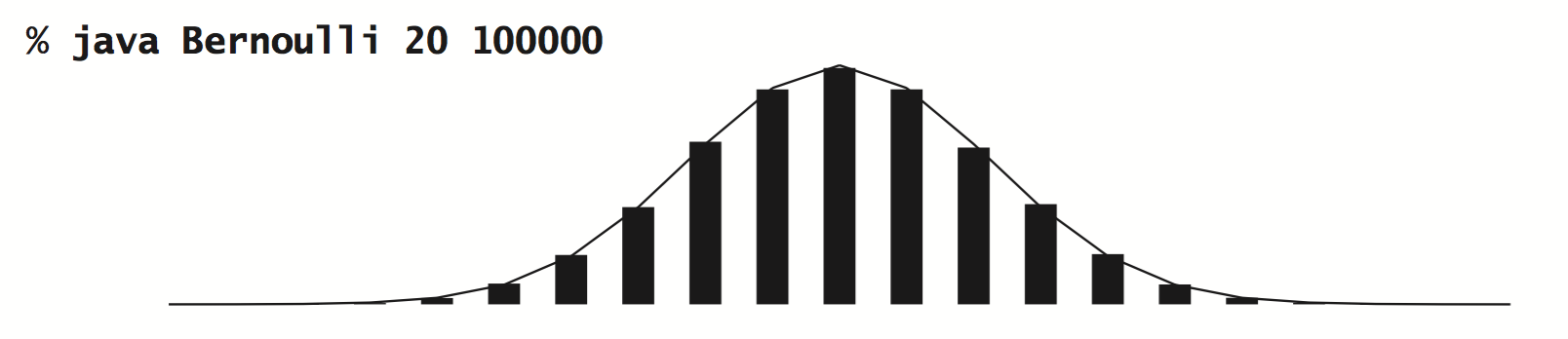Exercises

1. Add to Gaussian.java an implementation of the three-argument static method pdf(x, mu, sigma) specified in the API that computes the Gaussian probability density function with a given mean μ and standard deviation σ, based on the formula $$\phi(x, \mu, \sigma)$$ = $$\phi((x - \mu) / \sigma) / \sigma$$. Also add an implementation of the associated cumulative distribution function cdf(z, mu, sigma), based on the formula $$\Phi(z, \mu, \sigma)$$ = $$\Phi((z - \mu) / \sigma)$$.
2. Write a library of static method Hyperbolic.java that implements the hyperbolic functions based on the definitions $$\sinh(x) = (e^x - e^{-x}) / 2$$ and $$\cosh(x) = (e^x + e^{-x}) / 2$$, with $$\tanh(x)$$, $$\coth(x)$$, $$\text{sech}(x)$$, and $$\text{csch}(x)$$ defined in a manner analogous to standard trigonometric functions.
3. Add to StdRandom.java a method shuffle() that takes an array of double values as argument and rearranges them in random order. Implement a test client that checks that each permutation of the array is produced about the same number of times. Add overloaded methods that take arrays of integers and strings.
4. Develop a full implementation of StdArrayIO.java (implement all 12 methods indicated in the API).
5. Write a library Matrix.java that implements the following API:6. Write a Matrix.java client MarkovSquaring.java that implements the version of Markov.java described in Section 1.6 but is based on squaring the matrix, instead of iterating the vector–matrix multiplication.

Creative Exercises

1. Sicherman dice. Suppose that you have two six-sided dice, one with faces labeled 1, 3, 4, 5, 6, and 8 and the other with faces labeled 1, 2, 2, 3, 3, and 4. Write a program Sicherman.java to compare the probabilities of occurrence of each of the values of the sum of the dice with those for a standard pair of dice. Use StdRandom and StdStats.

Solution: dice with these properties are called Sicherman dice: they produce sums with the same frequency as regular dice (2 with probability 1/36, 3 with probability 2/36, and so on).

Web Exercises

1. Sample standard deviation. The sample standard deviation of a sequence of n observations is defined similar to the standard deviation except that we divide by n−1 instead of n. Add a method sampleStddev() that computes this quantity.
2. Barnsley fern. Write a program Barnsley.java that takes a command line argument N and plots a sequence of N points according to the following rules. Set (x, y) = (0.5, 0). Then update (x, y) to one of the following four quantities according to the probabilities given.

PROBABILITY NEW X NEW Y
2% 0.5 0.27y
15% -0.139x + 0.263y + 0.57 0.246x + 0.224y - 0.036
13% 0.170x - 0.215y + 0.408 0.222x + 0.176y + 0.0893
70% 0.781x + 0.034y + 0.1075 -0.032x + 0.739y + 0.27

The pictures below show the results after 500, 1000, and 10,000 iterations.3. Black-Scholes. The Black-Scholes model predicts that the asset price at time t will be S' = S exp { (rt - 0.5*sigma^2*t + sigma ε sqrt(t) }, where epsilon is a standard Gaussian random variable. Can use Monte Carlo simulate to estimate. To estimate the value of the option at time T, compute max(S' - X, 0) and take mean over many trials of epsilon. The value of the option today is e^-rT * mean. European put = max(X - S', 0). Reuse function. Name your program BlackScholes.java. See Exercise 2.1.30 for an exact formula for this case.
4. Simulation. Application: some kind of simulation which uses StdRandom and StdStats to flip coins and analyze mean/variance. [Ex: physics, financial based on Black-Scholes hedge simulation. Simulation needed to price options whose payoff depends on the price path, not just the price at the maturity time T. Ex: Asian average price call = max(0, S_bar - X) where S_bar is the average price of the asset from time 0 to T. Lookback option = max(0, S(T) - min_t S_t). Idea: discretize time into N periods.] another reference Break up simulation into various pieces encapsulated as functions.
5. Flaming fractals. Implement a generalization of IFS to produce fractal flames like Water Lilies by Roger Johnston. Flaming fractals differ from classic IFS by using nonlinear update functions (sinusoidal, spherical, swirl, horseshoe), using a log-density display to color pixels according to how many times they result in the process, and incorporating color based on which rule was applied to get to that point.
6. Random point on a sphere. Use StdRandom.gaussian() to generate a random point on the surface of a sphere or hypersphere using the following method: generate N random values from the gaussian distribution, x, ..., x[N-1]. Then (x/scale, ..., x[N-1]/scale) is a random point on the N-dimensional sphere, where scale = sqrt(x^2 + ... + x[N-1]^2).
7. Coupon collector. Write a modular program CouponExperiment.java that runs experiments to estimate the value of the quantity of interest in the coupon collector problem. Compare the experimental results from your program with the mathematical analysis, which says that the expected number of coupons collected before all N values are found should be about N times the Nth Harmonic number (1 + 1/2 + 1/3 + ... + 1/N) and the standard deviation should be about N π / sqrt(6).
8. Exponential distribution. Add a method exp() to StdRandom.java that takes an argument λ and returns a random number from the exponential distribution with rate λ. Hint: If x is a random number uniformly distributed between 0 and 1, then -ln x / λ is a random number from the exponential distribution with rate λ.